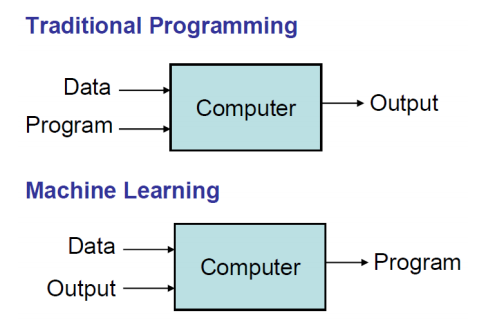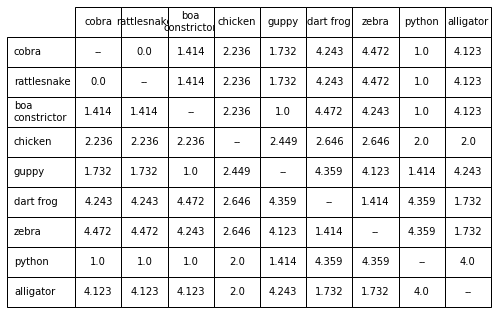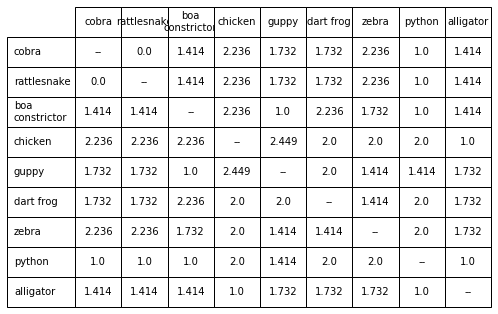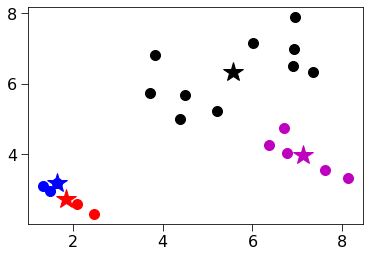• What is Machine Learning
• Many useful programs learn something
Note: "Field of study that gives computers the ability to learn without being explicitly programmed" - Arthur Samuel
- Modern statistics meets optimization• Observe set of examples: training data
• Infer something about process that generated that data
• Use inference to make predictions about previously unseen data: test data
• All ML Methods Require
• Representation of the features
• Distance metric for feature vectors
• Objective function and constraints
• Optimization method for learning the model
• Evaluation method
• Supervised Learning
• Goal : find a model that predicts a value for a previously unseen feature vector
• Regression models predict a real
• E.g. linear regression
• Classification models predict a label (chosen from a finite set of labels)
• Unsupervied Learning
• Goal : uncover some latent structure in the set of feature vectors
• Clustering the most common technique
• Define some metric that captures how similar one feature vector is to another
• Group examples based on this metric
• Choosing Features
• Features never fully describe the situation
• Feature Engineering
• Represent examples by feature vectors that will facilitate generalization
• Suppose I want to use 100 examples from past to predict which students will pass the final exam
• Some features surely helpful, e.g., their grade on the midterm, did they do the problem sets, etc.
• Others might cause me to overfit, e.g., birth month
• Whant to maximize ratio of useful input to irrelevant input
• Signal-to-Noise Ratio (SNR)
• K-Nearest Neighbors
• Distance between vectors
• Minkowski metric $$dist(X_1, X_2, p) = (\sum_{k=1}^{len}abs({X_1}_k - {X_2}_k)^p)^{\frac{1}{p}} \\ p=1 : \text{Manhattan Distance} \\ p=2 : \text{Euclidean Distance}$$
from lecture12_segment2 import *

cobra = Animal('cobra', [1,1,1,1,0])
rattlesnake = Animal('rattlesnake', [1,1,1,1,0])
boa = Animal('boa\nconstrictor', [0,1,0,1,0])
chicken = Animal('chicken', [1,1,0,1,2])
alligator = Animal('alligator', [1,1,0,1,4])
dartFrog = Animal('dart frog', [1,0,1,0,4])
zebra = Animal('zebra', [0,0,0,0,4])
python = Animal('python', [1,1,0,1,0])
guppy = Animal('guppy', [0,1,0,0,0])
animals = [cobra, rattlesnake, boa, chicken, guppy,
dartFrog, zebra, python, alligator]

compareAnimals(animals, 3) # k=3

Help on method scale in module matplotlib.table:

scale(xscale, yscale) method of matplotlib.table.Table instance
Scale column widths by *xscale* and row heights by *yscale*.• Using Distance Matrix for classification
• Simplest approach is probably nearest neighbor
• Remember training data
• When predicting the label of a new example
• Find the nearest example in the training data
• Predict the label associated with that example
• Learning fase, no explicit training
• No theory required
• Easy to explain method and results
• Memory intensive and predictions can take a long time
• Are better algorithms than brute force
• No model to shed light on process that generated data
cobra = Animal('cobra', [1,1,1,1,0])
rattlesnake = Animal('rattlesnake', [1,1,1,1,0])
boa = Animal('boa\nconstrictor', [0,1,0,1,0])
chicken = Animal('chicken', [1,1,0,1,2])
alligator = Animal('alligator', [1,1,0,1,1])
dartFrog = Animal('dart frog', [1,0,1,0,1])
zebra = Animal('zebra', [0,0,0,0,1])
python = Animal('python', [1,1,0,1,0])
guppy = Animal('guppy', [0,1,0,0,0])
animals = [cobra, rattlesnake, boa, chicken, guppy,
dartFrog, zebra, python, alligator]

compareAnimals(animals, 3) k = 3

Help on method scale in module matplotlib.table:

scale(xscale, yscale) method of matplotlib.table.Table instance
Scale column widths by *xscale* and row heights by *yscale*.• A more General Approach: Scaling
• Z-scaling
• Each feature has a mean of 0 & a standard deviation of 1
• Interpolation
• Map minimum value to 0, maximum value to 1, and linearly interpolate python def zScaleFeatures(vals): """Assumes vals is a sequence of floats""" result = np.array(vals) mean = np.mean(vals) result = result - mean return result/np.std(result)

def iScaleFeatures(vals): """Assumes vals is a sequence of floats""" minVal, maxVal = min(vals), max(vals) fit = np.polyfit([minVal, maxVal], [0, 1], 1) return np.polyval(fit, vals) 

• Clustering
• Partition examples into groups (clusters) such that examples in a group are more similar to each other than to examples in other groups
• Unlike classification, there is not typically a "right answer"
• Answer dictated by feature vector and distance metric, not by a group truth label
• Optimization Problem $$variability(c) = \sum_{e \in c} distance(mean(c), e)^2 \\ dissimilarity(C) = \sum_{c \in C} variability(c) \\ c :\text{one cluster} \\ C : \text{all of the clusters}$$
• Why not divide variability by size of cluster?
• Is optimization problem finding a $C$ that minimizes $dissimilarity(C)$?
• No, otherwise could put each example in its own cluster
• Need constraints, e.g.
• Minimum distance between clusters
• Number of clusters
• K-means Clustering
• Constraint: exactly k non-empty clusters
• Use a greedy algorithm to find an approximation to minimizing objective function
• Algorithm
randomly chose k examples as initial centroids
while true:
create k clusters by assigning each example to closest centroid
compute k new centroids by averaging examples in each cluster
if centroids dont change:
break
from lecture12_segment3 import *

centers = [(2, 3), (4, 6), (7, 4), (7,7)]
examples = []
random.seed(0)
for c in centers:
for i in range(5):
xVal = (c + random.gauss(0, .5))
yVal = (c + random.gauss(0, .5))
name = str(c) + '-' + str(i)
example = Example(name, pylab.array([xVal, yVal]))
examples.append(example)

xVals, yVals = [], []
for e in examples:
xVals.append(e.getFeatures())
yVals.append(e.getFeatures())

random.seed(2)
kmeans(examples, 4, True)

Iteration #1
Cluster color = 0
Cluster with centroid [1.66014278 3.18525178] contains:
(2, 3)-1
Cluster color = 1
Cluster with centroid [1.8494407 2.7367613] contains:
(2, 3)-0, (2, 3)-2, (2, 3)-3, (2, 3)-4
Cluster color = 2
Cluster with centroid [5.57612073 6.33385138] contains:
(4, 6)-0, (4, 6)-1, (4, 6)-2, (4, 6)-3, (4, 6)-4, (7, 7)-0, (7, 7)-1, (7, 7)-2, (7, 7)-3, (7, 7)-4
Cluster color = 3
Cluster with centroid [7.11402489 3.98797723] contains:
(7, 4)-0, (7, 4)-1, (7, 4)-2, (7, 4)-3, (7, 4)-4

Iteration #2
Cluster color = 0
Cluster with centroid [1.49914988 3.08204521] contains:
(2, 3)-1, (2, 3)-2, (2, 3)-4
Cluster color = 1
Cluster with centroid [2.28022797 2.44308067] contains:
(2, 3)-0, (2, 3)-3
Cluster color = 2
Cluster with centroid [5.57612073 6.33385138] contains:
(4, 6)-0, (4, 6)-1, (4, 6)-2, (4, 6)-3, (4, 6)-4, (7, 7)-0, (7, 7)-1, (7, 7)-2, (7, 7)-3, (7, 7)-4
Cluster color = 3
Cluster with centroid [7.11402489 3.98797723] contains:
(7, 4)-0, (7, 4)-1, (7, 4)-2, (7, 4)-3, (7, 4)-4

Iteration #3
Cluster color = 0
Cluster with centroid [1.49914988 3.08204521] contains:
(2, 3)-1, (2, 3)-2, (2, 3)-4
Cluster color = 1
Cluster with centroid [2.28022797 2.44308067] contains:
(2, 3)-0, (2, 3)-3
Cluster color = 2
Cluster with centroid [5.57612073 6.33385138] contains:
(4, 6)-0, (4, 6)-1, (4, 6)-2, (4, 6)-3, (4, 6)-4, (7, 7)-0, (7, 7)-1, (7, 7)-2, (7, 7)-3, (7, 7)-4
Cluster color = 3
Cluster with centroid [7.11402489 3.98797723] contains:
(7, 4)-0, (7, 4)-1, (7, 4)-2, (7, 4)-3, (7, 4)-4


[<lecture12_segment3.Cluster at 0x227612ac8c8>,
<lecture12_segment3.Cluster at 0x227612ac408>,
<lecture12_segment3.Cluster at 0x227612ac588>,
<lecture12_segment3.Cluster at 0x227612ac6c8>]• Mitigating Dependence on Initial Centroids
best = kMeans(points)
for t in range(numTrials):
C = kMeans(points)
if dissimilarity(C) < dissimilarity(best):
best = C
return best
`
• A Pretty Example
• User k-means to cluster groups of pixels in an image by their color
• Get the color associated with the centroid of each cluster, i.e., the average color of the cluster
• For each pixel in the original image, find the centroid that is its nearest neighbor
• Replaced the pixel by that centroid